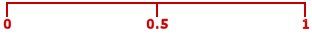Go to contentProbability is a way of assessing chance, likelihood or risk. It may be quoted as a decimal, fraction or percentage. The probability of an impossible event is 0 and the probability of a certain event is 1. All other probabilities are between 0 and 1.You can get a better display of the maths by downloading special TeX fonts from jsMath. In the meantime, we will do the best we can with the fonts you have, but it may not be pretty and some equations may not be rendered correctly.

## Glossary

### event

any set of possible outcomes of a statistical experiment

### fraction

A ratio of two numbers or polynomials.

### union

The union of two sets A and B is the set containing all the elements of A and B.

Full Glossary List

## This question appears in the following syllabi:

SyllabusModuleSectionTopicExam Year
AQA GCSE (9-1) Foundation (UK)P: ProbabilityP1: Frequencies in Tables and TreesBasic Probability Problems-
CBSE IX (India)ProbabilityExperimentsRepeated experiments-
CBSE X (India)Statistics and ProbabilityProbabilityDefinition-
CIE IGCSE (9-1) Maths (0626 UK)8 ProbabilityC8.1 Calculating a ProbabilityBasic Probability Problems-
Edexcel GCSE (9-1) Foundation (UK)P: ProbabilityP1: Frequencies in Tables and TreesBasic Probability Problems-
GCSE Foundation (UK)ProbabilityProbabilityBasic problems-
OCR GCSE (9-1) Foundation (UK)11: Probability11.01d: Equally Likely Outcomes and ProbabilityBasic Probability Problems-
Universal (all site questions)PProbabilityBasic problems-